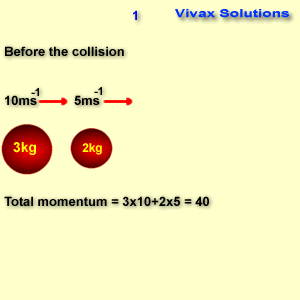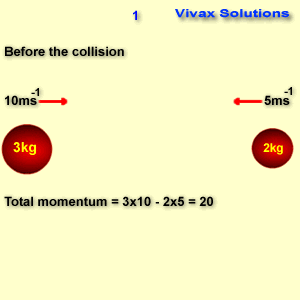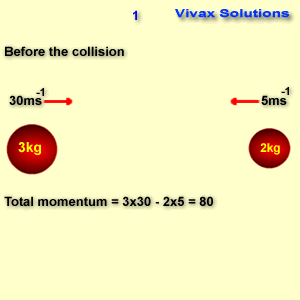# Mathematics

#### Linear Momentum, Conservation of Linear Momentum and Impulse

In this tutorial, you will learn the following:

• Linear momentum
• The principle of conservation of linear momentum
• Impulse
• Worked examples involving conservation of momentum and impulse

#### Linear Momentum

The product of mass and velocity is called momentum.
It is a vector - has both magnitude and direction - and its units are kgms-1.
momentum = mass x velocity
momentum = mv

E.g.1

The mass of an object is 5kg and velocity is 2 ms-1. Find its momentum.
momentum = 5 x 2 = 10 kgms-1

E.g.2

The mass of an object is 2 kg. It moves towards a wall at 20 ms-1, hits the wall and bounces back at 10 ms-1. Find the change in momentum.
momentum towards the wall = 2 x 20 = 40 kgms-1
momentum away from the wall after the impact = 2 x 10 = 20 kgms-1
Change in momentum = 40 - -20 = 60 kgms-1 towards the wall.

#### The Principle of Conservation of Linear Momentum

When two of more objects collide, the total momentum, before and after the collision, remains the same, in the absence of external forces.

Case 1

Two objects of mass 3 kg an 2 kg move at 10 ms-1 and 5ms-1 respectively in the same direction. After the impact, they move as a single combined object. Find its speed.Case 2

Two objects of mass 3 kg an 2 kg move at 10 ms-1 and 5ms-1 respectively in the opposite directions. After the impact, they move as a single combined object. Find its speed.Case 3

Two objects of mass 3 kg an 2 kg move at 30 ms-1 and 5ms-1 respectively in the opposite directions. After the impact, the heavier object reverses the direction at 10 ms-1. Find the speed of the other object and the direction.#### Linear Momentum and Explosions

The principle of linear momentum can be applied during explosions too. For example, it can be used when a gun fires a bullet or a firecracker explodes. We, however, have to assume that their is no loss of mass during the events.

E.g.1

The mass of a gun is 12kg and it fires a bullet of mass 40g at 200 m/s. Why does the gun recoil? Find the recoiling speed of the gun too.

The total momentum before the bullet being fired = 0 kgm/s
Since the bullet moves forward after being fired, the gun has to go backwards to make the total momentum after the bullet being fired is equal to zero - the principal conservation of linear momentum
If the velocity of the gun backwards is v,
12v - 0.04 x 200 = = 0
v = 8/12 = 0.6 m/s
The gun recoils at a relatively smaller speed due to its higher mass.

E.g.2

A firecracker of mass 20 g explodes into two parts; the heavier of the two, 12g, moves at 30 m/s. Find the velocity of the smaller part.
The total momentum before the explosion = 0
If the velocity of the lighter part is v,
The total momentum after the explosion = 12 x 30 - 8v
According to the principle of conservation of linear momentum,
8v - 360 = 0
v = 45 m/s.

#### Impulse(I)

The product of the force and the time it acts on an object is called impulse.

Units: Ns
Impulse is a vector.

I = Ft
Since F = ma
I = mat
= m(v-u)/t x t
= m(v-u)
= mv - mu
I = mv - mu
The impulse is equal to the change in momentum.

E.g. 1

The mass of an object is 2kg and is moving at 20 m/s. If a force is applied on it, its velocity changes to 30 m/s. Find the impulse by the force on the object. It it acts on the object for 0.1s, find the force as well.

I = mv - mu
2x30 - 2x20 = 20 Ns
I = Ft
20 = F x 0.1
F = 200N.

E.g. 2

The mass of a ball is 5kg and its hits a wall horizontally at 40 m/s and then bounces back at 10 m/s. Find the impact on the wall. Hence, find the impact on the ball by the wall too.

I = mv - mu
= 5x40 - - 5x10
= 200 + 50
= 250 Ns
The impact on the ball is the same as the impact on the wall - Newton's Third Law
The direction of the impulse on the the ball, however, is in the opposite direction.

#### ChallengeA man is standing inside his boat, which remains stationary on still water in a lake, at the far-end. He intends to walk to the opposite end in the hope of getting to the shore as close as possible. Will it work? Explain the answer. If the mass of the man and the boat are 60kg and 300kg respectively, find by how much he gets closer or further from the shore. The length of the boat and the walking speed of the man, relative to the boat, are 4m and 2m/s respectively.

#### Practice Questions

Now work out the following to complement what you have learnt so far.

1. Masses of two object are 3 kg and 2 kg respectively. They move in opposite directions at 20 ms-1 and 2 ms-1 respectively. After the collision, the lighter object reverses its motion with the speed being the same. Determine the subsequent motion of the heavier object.
2. Three spheres of the same radii are placed in a straight line on a smooth table. Their masses are 2kg, 5kg and 6kg respectively. The first one moves at 'u' ms-1 and hits the second one. On impact, the speed of the second one becomes 'u'. Then the second one hits the third one, and on impact, the speed of the third one becomes 'u'. Find whether the first one and the second one would collide again.
3. The mass of an object is 2 kg. It moves at 20 ms-1 and hits a wall. After 0.5 seconds, it reverses the direction at 5 ms-1. Find the change in momentum and hence calculate the force exerted on the wall.
4. Two objects of mass 2 kg and 5 kg move towards each other at 20ms-1 and 10 ms-1 respectively. After the impact, they move as a single object. Find its speed.
5. A wooden block of mass 3kg is suspended by a string on a ceiling. A bullet of mass 15g hits the block horizontally and moves through gets embedded in it. Subsequently, the block moves through a vertical distance of 10cm. Find the speed of the bullet.
6. A bullet is fired from a gun with a velocity of 1000 m/s. The gun recoils with velocity 2 m/s. What is the ratio of the masses of the gun and the bullet?
7. A grenade of mass 3kg explodes into two parts. The lighter part of mass 1kg moves at 60m/s. Find the speed of the heavier part.
8. A bullet of mass 20g, moving at 200 m/s, hits a sack of sand of mass 20kg. Find the speed at which the system slips along the ground of coefficient of friction 0.4.
9. Two objects of mass 5kg, each, are connected to a compressed spring and placed on a smooth plane. One of them is then released at 10m/s. Find the speed of the system after being released, stating your assumptions.
10. A meteorite burns out in the atmosphere before it collides with the Earth's surface. How do you account for the loss of its momentum?

Move the mouse over, just below this, to see the answers:

1. 17.3
2. No
3. 100
4. -1.4
5. 2814
6. 1:500
7. 30
8. 0.005
9. 5
10. Explain it.Maths is challenging; so is finding the right book. K A Stroud, in this book, cleverly managed to make all the major topics crystal clear with plenty of examples; popularity of the book speak for itself - 7th edition in print.

### Recommended - GCSE & iGCSEThis is the best book available for the new GCSE(9-1) specification and iGCSE: there are plenty of worked examples; a really good collection of problems for practising; every single topic is adequately covered; the topics are organized in a logical order.

### Recommended for A LevelThis is the best book that can be recommended for the new A Level - Edexcel board: it covers every single topic in detail;lots of worked examples; ample problems for practising; beautifully and clearly presented.